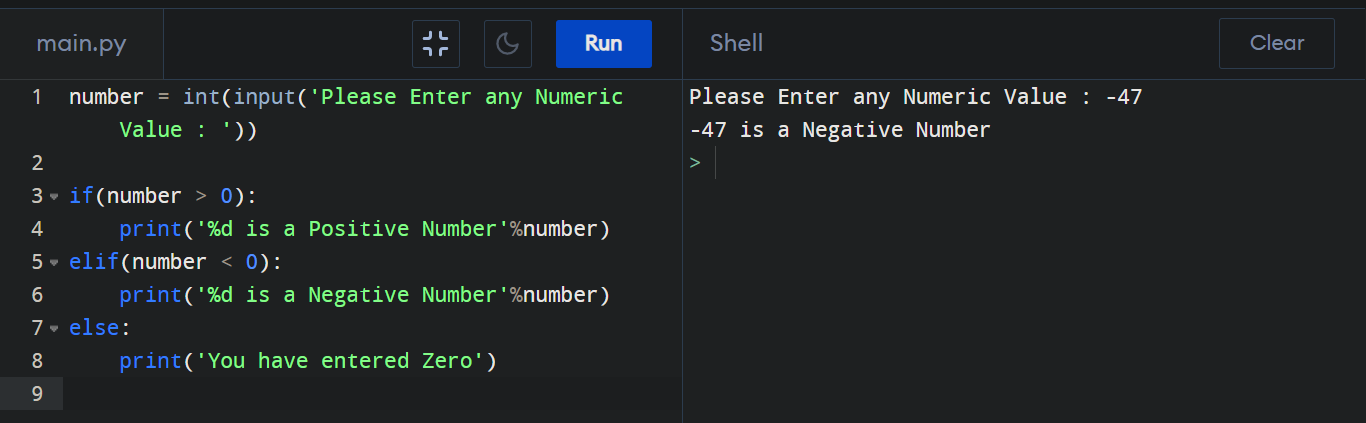## Python Program to check Number (Positive or Negative or Zero)

How to check if a Number is Positive or Negative or Zero? By this following program we can do this. Here we used "if-else" statements to check the Number type.

#### Positive or Negative checking by Python :

``````
number = int(input('Please Enter any Numeric Value : '))

if(number > 0):
print('%d is a Positive Number'%number)
elif(number < 0):
print('%d is a Negative Number'%number)
else:
print('You have entered Zero')

``````

#### Output :